Tamilnadu Samacheer Kalvi 6th Maths Solutions Term 2 Chapter 1 Numbers Ex 1.1

Question 1.
Fill in the blanks.
(i) The number of prime numbers between 11 and 60 is _______
(ii) The numbers 29 and _______ are twin primes.
(iii) 3753 is divisible by 9 and hence divisible by _______
(iv) The number of distinct prime factors of the smallest 4 digit number is______
(v) The sum of distinct prime factors of 30 is ________
Solution:
(i) 12
(ii) 31
(iii) 3
(iv) 2
(v) 10

Question 2.
Say True or False
(i) The sum of any number of odd numbers is always even.
(ii) Every natural number is either prime or composite.
(iii) If a number is divisible by 6, then it must be divisible by 3.
(iv) 16254 is divisible by each of 2, 3, 6 and 9.
(v) The number of distinct prime factors of 105 is 3.
Solution:
(i) False
(ii) False
(iii) True
(iv) True
(v) True

Question 3.
Write the smallest and the biggest two digit prime number.
Solution:
Smallest is 11
Biggest is 97

Question 4.
Write the smallest and the biggest three-digit composite number.
Solution:
Smallest three-digit composite number – 100
Biggest three-digit composite number – 999

Question 5.
The sum of any three odd natural numbers is odd. Justify this statement with an example.
Solution:
True, as we know, that,“the sum of any three odd numbers is always an odd number”.
Example: 3 + 7 + 9 = 19 is odd.Question 6.
The digits of the prime number 13 can be reversed to get another prime number 31. Find if any such pairs exist up to 100.
Solution:
(17, 71), (37, 73) and (79, 97)

Question 7.
Your friend says that every odd number is prime. Give an example to prove him/her wrong.
Solution:
15 is an odd number not prime.

Question 8.
Each of the composite numbers has at least three factors. Justify this statement with an example.
Solution:
True. The composite number 4 has 3 factors namely 1, 2 and 4.

Question 9.
Find the dates of any month of a calendar which are divisible by both 2 and 3.
Solution:
Every month the dates 6, 12, 18, 24 and 30 (excluding February) are divisible by both 2 and 3.

Question 10.
I am a two-digit prime number and the sum of my digits is 10.1 am also one of the factors of 57. Who am I?
Solution:
19Question 11.
Find the prime factorisation of each number by factor tree method and division method.
(a) 60
(b) 128
(c) 144
(d) 198
(e) 420
(f) 999
Solution:
(a) 60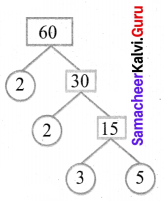∴ 60 = 2 × 2 × 3 × 5
Also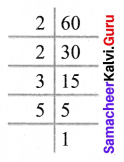∴ 60 = 2 × 2 × 3 × 5
(b) 128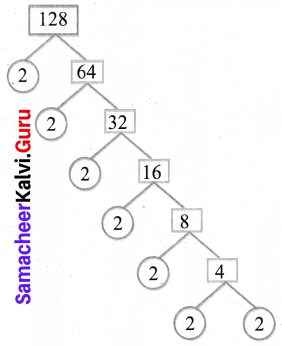∴ 128 = 2 × 2 × 2 × 2 × 2 × 2 × 2
Also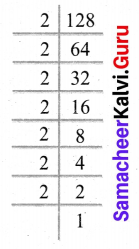∴ 128 = 2 × 2 × 2 × 2 × 2 × 2 × 2
(c) 144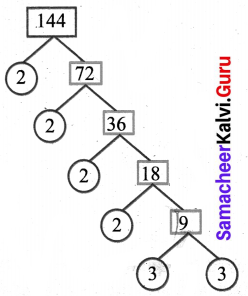∴ 144 = 2 × 2 × 2 × 2 × 3 × 3
Also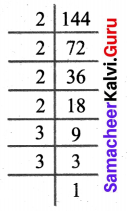∴ 144 = 2 × 2 × 2 × 2 × 3 × 3
(d) 198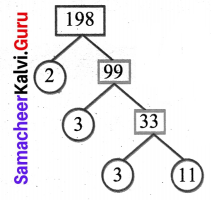∴ 198 = 2 × 3 × 3 × 11
Also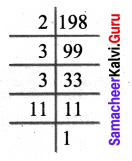∴ 198 = 2 × 3 × 3 × 11
(e) 420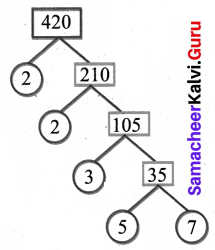∴ 420 = 2 × 2 × 3 × 5 × 7
Also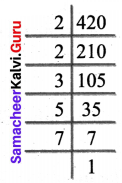∴ 420 = 2 × 2 × 3 × 5 × 7
(f) 999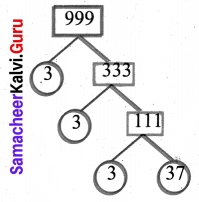∴ 999 = 3 × 3 × 3 × 37
Also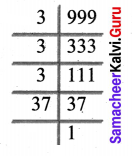∴ 999 = 3 × 3 × 3 × 37Question 12.
If there are 143 math books to be arranged in equal numbers in all the stacks, then find the number of books in each stack and also the number of stacks.
Solution:
Total number of books = 143
Factorizing 143 = 11 × 13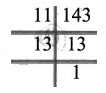A number of stacks and number of books in each stack may be (11, 13) or (13, 11).

Objective Type Questions

Question 13.
The difference between two successive odd number is
(a) 1
(b) 2
(c) 3
(d) 0
Solution:
(b) 2

Question 14.
The only even prime number is
(a) 4
(b) 6
(c) 2
(d)
Solution:
(c) 2

Question 15.
Which of the following numbers is not prime?
(a) 53
(b) 92
(c) 97
(d) 71
Solution:
(b) 92

Question 16.
The sum of the factors of 27 is
(a) 28
(b) 37
(c) 40
(d) 31
Solution:
(c) 40

Question 17.
The factors of a number are 1, 2, 4, 5, 8, 10, 16, 20, 40 and 80. What is the number?
(a) 80
(b) 100
(c) 128
(d) 160
Solution:
(a) 80

Question 18.
The prime factorisation of 60 is 2 × 2 × 3 × 5. Any other number which has the same prime factorisation as 60 is
(a) 30
(b) 120
(c) 90
(d) impossible
Solution:
(d) impossibleQuestion 19.
If the number 6354*97 is divisible by 9, then the value * is
(a) 2
(b) 4
(c) 6
(d) 7

Solution:
(a) 2

Question 20.
The number 87846 is divisible by
(a) 2 only
(b) 3 only
(c) 11 only
(d) all of these
Solution:
(d) all of these